# The NLMIXED Procedure

### Prediction

The nonlinear mixed model is a useful tool for statistical prediction. Assuming a prediction is to be made regarding the ith subject, suppose that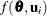is a differentiable function predicting some quantity of interest. Recall thatdenotes the vector of unknown parameters and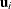denotes the vector of random effects for the ith subject. A natural point prediction is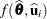, where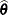is the maximum likelihood estimate ofand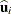is the empirical Bayes estimate ofdescribed previously in the section Integral Approximations.

An approximate prediction variance matrix for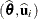is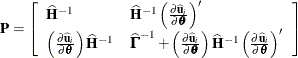where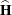is the approximate Hessian matrix from the optimization for,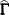is the approximate Hessian matrix from the optimization for, and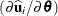is the derivative ofwith respect to, evaluated at. The approximate variance matrix foris the standard one discussed in the previous section, and that foris an approximation to the conditional mean squared error of prediction described by Booth and Hobert (1998).

The prediction variance for a general scalar functionis defined as the expected squared difference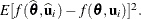PROC NLMIXED computes an approximation to it as follows. The derivative ofis computed with respect to each element of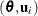and evaluated at. If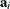is the resulting vector, then the approximate prediction variance is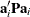. This approximation is known as the delta method (Billingsley, 1986; Cox, 1998).